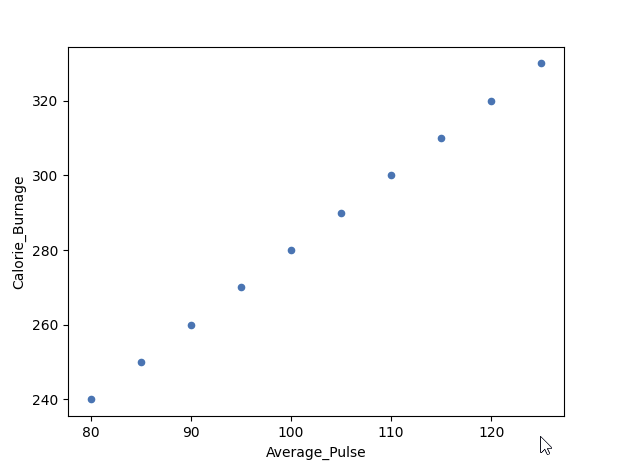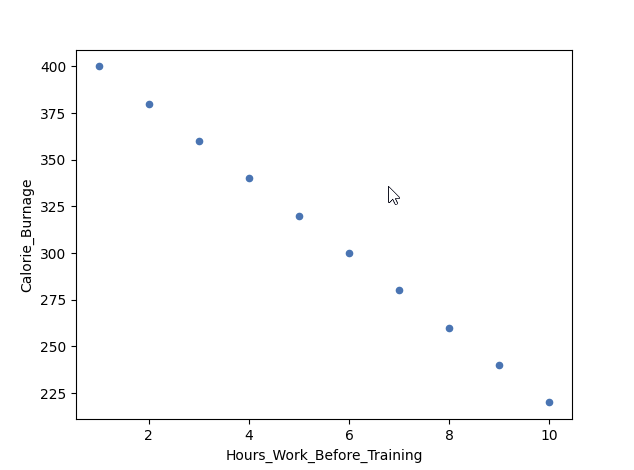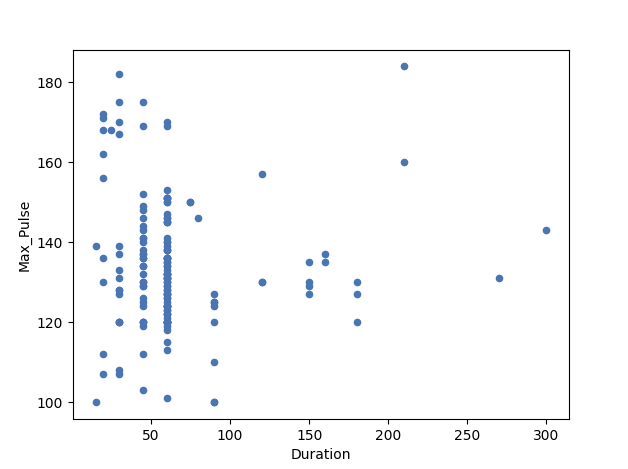# Data Science - Statistics Correlation

## Correlation

Correlation measures the relationship between two variables.

We mentioned that a function has a purpose to predict a value, by converting input (x) to output (f(x)). We can say also say that a function uses the relationship between two variables for prediction.

## Correlation Coefficient

The correlation coefficient measures the relationship between two variables.

The correlation coefficient can never be less than -1 or higher than 1.

• 1 = there is a perfect linear relationship between the variables (like Average_Pulse against Calorie_Burnage)
• 0 = there is no linear relationship between the variables
• -1 = there is a perfect negative linear relationship between the variables (e.g. Less hours worked, leads to higher calorie burnage during a training session)

## Example of a Perfect Linear Relationship (Correlation Coefficient = 1)

We will use scatterplot to visualize the relationship between Average_Pulse and Calorie_Burnage (we have used the small data set of the sports watch with 10 observations).

This time we want scatter plots, so we change kind to "scatter":

### Example

import matplotlib.pyplot as plt

health_data.plot(x ='Average_Pulse', y='Calorie_Burnage', kind='scatter')
plt.show()
Try it Yourself »

Output:As we saw earlier, it exists a perfect linear relationship between Average_Pulse and Calorie_Burnage.

## Example of a Perfect Negative Linear Relationship (Correlation Coefficient = -1)We have plotted fictional data here. The x-axis represents the amount of hours worked at our job before a training session. The y-axis is Calorie_Burnage.

If we work longer hours, we tend to have lower calorie burnage because we are exhausted before the training session.

The correlation coefficient here is -1.

### Example

import pandas as pd
import matplotlib.pyplot as plt

negative_corr = {'Hours_Work_Before_Training': [10,9,8,7,6,5,4,3,2,1],
'Calorie_Burnage': [220,240,260,280,300,320,340,360,380,400]}
negative_corr = pd.DataFrame(data=negative_corr)

negative_corr.plot(x ='Hours_Work_Before_Training', y='Calorie_Burnage', kind='scatter')
plt.show()
Try it Yourself »

## Example of No Linear Relationship (Correlation coefficient = 0)Here, we have plotted Max_Pulse against Duration from the full_health_data set.

As you can see, there is no linear relationship between the two variables. It means that longer training session does not lead to higher Max_Pulse.

The correlation coefficient here is 0.

### Example

import matplotlib.pyplot as plt

full_health_data.plot(x ='Duration', y='Max_Pulse', kind='scatter')
plt.show()
Try it Yourself »

W3Schools is optimized for learning and training. Examples might be simplified to improve reading and learning. Tutorials, references, and examples are constantly reviewed to avoid errors, but we cannot warrant full correctness of all content. While using W3Schools, you agree to have read and accepted our terms of use, cookie and privacy policy.